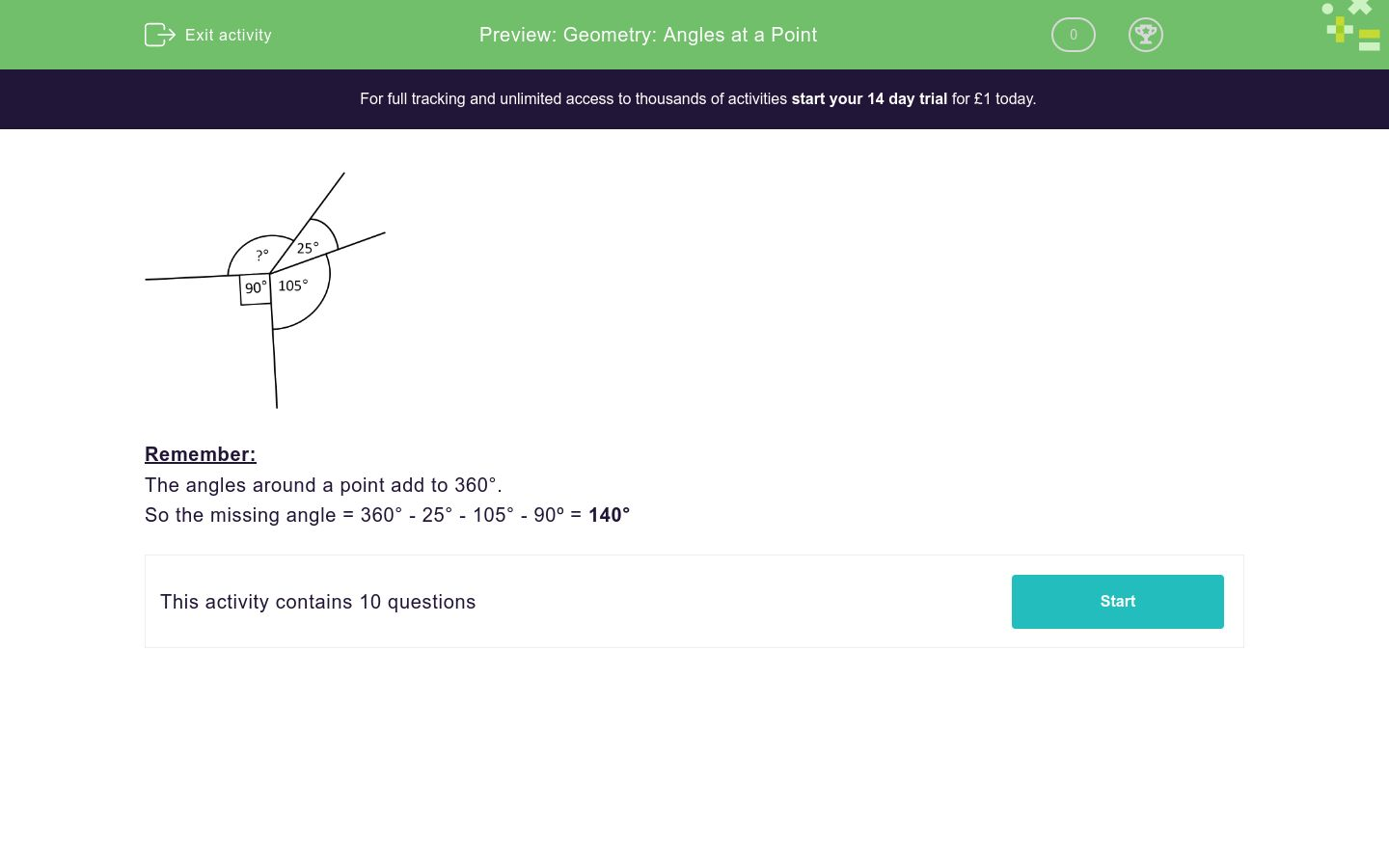# Geometry: Angles at a Point

In this worksheet, students calculate the missing angle around a point.Key stage:  KS 2

Curriculum topic:   Geometry: Properties of Shapes

Curriculum subtopic:   Recognise/Find Angles

Difficulty level:### QUESTION 1 of 10Remember:

The angles around a point add to 360°.

So the missing angle = 360° - 25° - 105° - 90º = 140°

---- OR ----